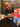# JavaScript Basics - this

September 03, 2020

## How `this` works in our code bases ?

Its is based on where we call and how we call

``````let a = {
b: 6,
c: 7,
d: function() {
return this.b + this.c;
}
}
a.d();
let f = a.d;
f();
window.b = 6;
window.c = 8;
f();``````

## When do we use `this` in our code bases ?

### Sample Code using this for variables

We use the `this` keyword in cases when we would like to pass the data in the constructor and use that variables in other functions. In the below example we are passing `a` and `b` variables. This variables are used in all functions.

``````class Sample {
constructor(a, b) {
this.a = a;
this.b = b;
}
sum() {
return this.a + this.b;
}

diff() {
return this.a - this.b;
}

multiply() {
return this.a * this.b;
}
}
let sam = new Sample(5,6);
let sum = sam.sum();
let diff = sam.diff();
let multiply = sam.multiply();``````

### Sample Code using this for functions

We use `this` in cases when we want to access functions from other functions. There is no confusion here till now.

``````class Sample {
constructor(a, b) {
this.a = a;
this.b = b;
}
sum() {
return this.a + this.b;
}

diff() {
return this.a - this.b;
}

multiply() {
return this.a * this.b;
}
printAll(){
console.log(this.sum())
console.log(this.diff())
console.log(this.multiply())
}
}
let sam = new Sample(5,6);
let sum = sam.printall();
let diff = sam.diff();
let multiply = sam.multiply();``````

### Sample Code using this for map

This below code throws an error. Why ? This is because we are using passing the callback function in foreach. This parameter function is not is binded to any function and executed some where else.

This is the reason we use bind. Bind will tell the function what is the `this` means.

``````class SampleMapExample {
constructor(a, b) {

this.arr = [1 , 2, 3];
this.sum = 0;
}